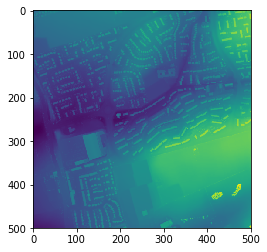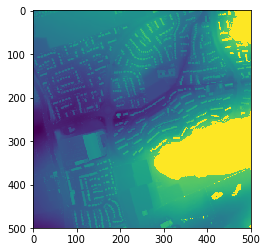# ENVI binary to GeoTiff - opening, processing and output in Python

Based on a post from a few years ago, a few people have asked how to handle ENVI format files in python for processing with the end goal being to output them as GeoTiff files. Purely for file format transformation, the easiest way for me is to use GDAL directly e.g. to convert a ENVI binary file (with a header file) to a GeoTIFF:

``````gdal_translate -of GTiff input_file.bin output_file.tif
``````

If you want to do the file reading, data manipulation and output all within python, then read on. The below provides some wrapper functions collating a number of gdal-python specific commands. I’m not going into the details as to exactly what is happening - have a look at the comments within the function code below if you’re interested.

So, without further ado, lets say we have some ENVI format binary data - we’ll use a sample of the OGL EA data in the UK converted for the purposes of this work from here. Download the binary file and the header file and make sure they are in the same directory.

What we want to do is:

1. Open the data in Python
2. Convert it to a numpy array
3. Do some processing (change the numbers)
4. Write it out as a geotiff

To do this, we’ll use the gdal bindings. So start with the following imports (if you need to install them look here):

``````import sys
import os
from osgeo import gdal, gdalconst
from osgeo.gdalconst import *
``````

To open the data, use the following:

``````def load_data(file_name, gdal_driver='GTiff'):
'''
Converts a GDAL compatable file into a numpy array and associated geodata.
The rray is provided so you can run with your processing - the geodata consists of the geotransform and gdal dataset object
If you're using an ENVI binary as input, this willr equire an associated .hdr file otherwise this will fail.
This needs modifying if you're dealing with multiple bands.

VARIABLES
file_name : file name and path of your file

RETURNS
image array
(geotransform, inDs)
'''
driver = gdal.GetDriverByName(gdal_driver) ## http://www.gdal.org/formats_list.html
driver.Register()

if inDs is None:
print("Couldn't open this file: %s" %(file_name))
print('/nPerhaps you need an ENVI .hdr file? A quick way to do this is to just open the binary up in ENVI and one will be created for you.')
sys.exit("Try again!")
else:
print("%s opened successfully" %file_name)

# Extract some info form the inDs
geotransform = inDs.GetGeoTransform()

# Get the data as a numpy array
band = inDs.GetRasterBand(1)
cols = inDs.RasterXSize
rows = inDs.RasterYSize
image_array = band.ReadAsArray(0, 0, cols, rows)

return image_array, (geotransform, inDs)
``````

This returns a numpy array of the data (`data`) as well as a tuple of data relating to the dataset your read in including coordinate info (`geodata`). Let’s open and plot the numpy array output to see that it looks as expected:

``````file_name="sk5545_DSM_2M.bin"

import matplotlib.pyplot as plt
plt.imshow(data)
``````Now we’ll process the data we’ve opened as a numpy array - let’s just change the numbers a bit and set anything bigger than 90 to 100:

``````new_data=data
new_data[data>90]=100
``````

Let’s plot it to see that it looks as expected/is different:

``````plt.imshow(data2)
``````Next we need to wrote it out so let’s rewrite a new function:

``````def array2raster(data_array, geodata, file_out, gdal_driver='GTiff'):
'''
Converts a numpy array to a specific geospatial output
If you provide the geodata of the original input dataset, then the output array will match this exactly.
If you've changed any extents/cell sizes, then you need to amend the geodata variable contents (see below)

VARIABLES
data_array = the numpy array of your data
geodata = (geotransform, inDs) # this is a combined variable of components when you opened the dataset
geotransform = inDs.GetGeoTransform()
see data2array()
file_out = name of file to output to (directory must exist)
gdal_driver = the gdal driver to use to write out the data (default is geotif) - see: http://www.gdal.org/formats_list.html

RETURNS
None
'''

if not os.path.exists(os.path.dirname(file_out)):
print("No further processing will take place.")
else:
post=geodata
original_geotransform, inDs = geodata

rows, cols = data_array.shape
bands = 1

# Set the gedal driver to use
driver = gdal.GetDriverByName(gdal_driver)
driver.Register()

# Creates a new raster data source
outDs = driver.Create(file_out, cols, rows, bands, gdal.GDT_Float32)

originX = original_geotransform
originY = original_geotransform

outDs.SetGeoTransform([originX, post, 0.0, originY, 0.0, -post])
outDs.SetProjection(inDs.GetProjection())

#Write raster datasets
outBand = outDs.GetRasterBand(1)
outBand.WriteArray(data_array)

print("Output saved: %s" %file_out)
``````

Now call it: this takes in the new array (`new_data`) and the original `geodata` tuple. The `gdal_driver` option is important to note as this is specific for the format of the data you want to output - have a look here for other compatible formats.

``````file_out="./test.tif"
array2raster(new_data, geodata, file_out, gdal_driver='GTiff')
``````

Now stick it all together:

``````## Open some data
file_name="sk5545_DSM_2M.bin"

# Plot it
plt.imshow(data)

## Do some stuff on the data (here I just change the numbers a bit)
new_data=(data*10)/3.3

# Plot the new data
plt.imshow(new_data)

# Write it out as a geotiff
file_out="./test.tif"
array2raster(new_data, geodata, file_out, gdal_driver='GTiff')

# Check your output (have a look in QGIS or something)
## by file size...
if os.stat(file_out).st_size == 0:
print("Doesn't look like the file wrote out properly...")
else:
print("Output file contains something - plot it or check in GIS")
## or by using your function...